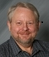## What is the formula to calculate the manpower requirement at a call center?Tags:
Call Center
Telecom
What is the formula to calculate the manpower requirement at a call center, if the no.of calls are specified for example, as 50000 calls and defined AHT is 150 secs per call?

Thanks. We'll let you know when a new response is added.

## What is the formula to calculate the manpower requirement at a call center?

Step1:
Calculate the call handling capacity of an executive (CHC)
CHC= (Staffing time * Occupancy%) / ACHT
For example if you have to calculate CHC of an executive with staffing time of 400 minutes/day and ACHT of 10 minutes, working at an occupancy of 80% then
CHC = (400 * 80%) / 10 = 32

So, an executive will be able to handle 32 calls in a day. Keep in mind, both Staffing Time and ACHT have to be in same time unit i.e. minutes or seconds.

Step 2:
Calculate required manpower in a day(RMP)
RMP= FC / CHC
For example if the daily forecast is 3200 calls and CHC is 32 calls then
RMP= 3200 / 32 = 100
So you will need 100 executives present to take calls to cater to this call volume.

This is a linear calculation which can be used for both inbound and outbound call centers.

## Discuss This Question: 10 Replies

Thanks. We'll let you know when a new response is added.
• What is the formula to calculate the manpower requirement at a call center, if the no.of calls are specified for example, as 50000 calls and defined AHT is 150 secs per call?
report
•Before we would want to give an answer to this, we would like to know do you have a business case for asking this question or is it a homework question.

If you have a good business case for it, please let us know. It is really not right for us to be doing homework for people. It deprives them of the extra knowledge they get when doing the research themselves.

report
• Staff time in mints * occupancy / acht
report
• report
• Devil need formal in excel if you could attach here.
report
• how about if total call received approximately 9000 calls in a month & its a 24/7 contact centre, how may headcount is required? when the peak hour is from 8am - 7pm
report
•@prabba08, here is a online calculator: https://planetcalc.com/3151/
report
• Formula is good for a learner .
You can mention it in one equation to save time.